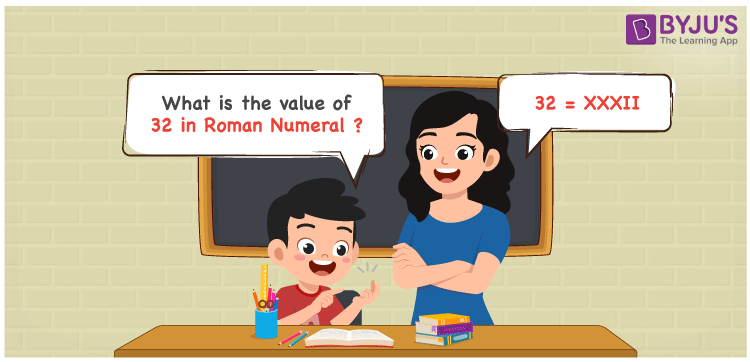# 32 in Roman Numerals

32 in Roman Numerals is XXXII. To represent any number in Roman numerals, the 7 primary symbols are used with certain rules. Refer to the rules of Roman numerals. 32 is represented as XXXII in the Roman numerals and it follows the addition rule. In the representation XXI, the numerals X is added thrice and the resultant is added to 2 times I.

 Number Roman Numeral 32 XXXII

## How to Write 32 in Roman Numerals?To convert 32 in Roman Numerals, we need to split the number according to the available symbols. i,e I, V, X, L, C, D, and M.

The simple way to split 32 will be 30 + 2. (But there are no primary letters for 30 and 2).

Hence 32 can be represented as 10 + 10 + 10 + 1 + 1. So

32 = 10 + 10 + 10 + 1 + 1

32 = X + X + X + I + I

32 = XXXII

## Video Lesson on Roman Numerals## Frequently Asked Questions on 32 in Roman Numerals

Q1

### How to write the number 32 in Roman Numerals?

32 in Roman Numerals is written as XXXII.
Q2

### Does XXI represent the number 32?

No. XXI represents the number 21 and not 32.
Q3

### Is XXIXXI a valid Roman numeral?

No. XXIXXI is not a valid Roman numeral.